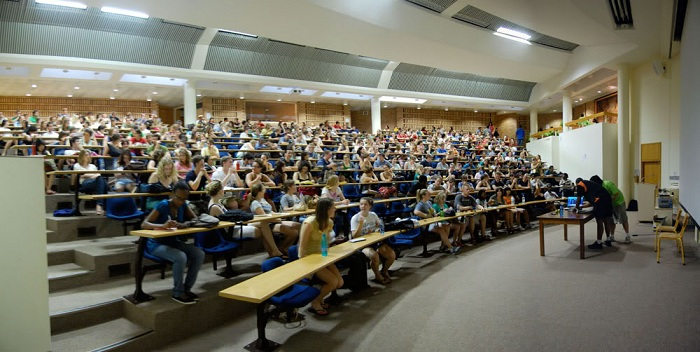### What is statistics?

Statistics is the scientific application of mathematical principles to the collection, analysis, and presentation of data. Statisticians contribute to scientific enquiry by applying their mathematical and statistical knowledge to the design of surveys and experiments; the collection, processing, and analysis of data; and the interpretation of the results.

### Who would be interested in studying statistics?

Statistics is a mathematical science, and so a taste and aptitude for mathematical thinking is a crucial ingredient. The field of statistics, like other areas of applied mathematics, often attracts those interested in the analysis of patterns in data: developing, understanding, abstracting, and packaging analytical methods for general use in other subject areas. Statistics is also, by definition, an information science. Imaginative use of both computing power and new computing environments drives much current research - so an interest in computation and/or computer science can also be a start for a statistician.